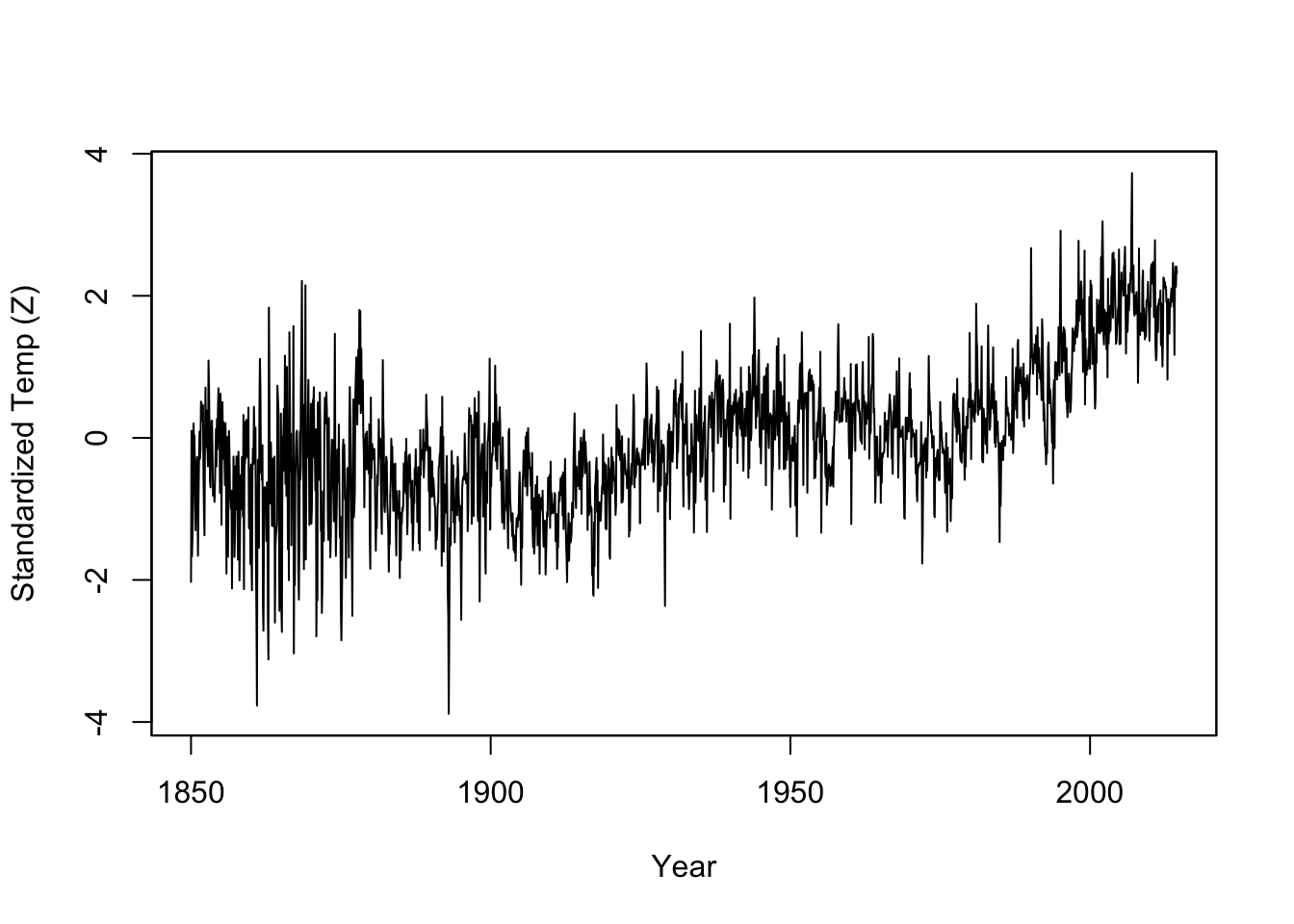# Time Series Definitions

Many ecological, epidemiological, and physical data records come in the form of time series. A time series is a sequence of observations recorded at a succession of time intervals.

In general, time series are characterized by dependence. The value of the series at some time $$t$$ is generally not independent of its value at, say, $$t-1$$. We use specialized statistics to analyze time series and specialized data structures to represent them in R. These data structures greatly facilitate our subsequent analysis.

These notes provide a very telegraphic introduction to some tools that I have found useful for disease ecology.

## Some Definitions

Time Series

A time series is a set of data indexed by time. For example $$\{y_t: t=1,2,\ldots n\}$$. Diggle (1990) notes that observations do not need to be evenly spaced and that a “more honest” notation might be $$\{y(t_i): t=1,2,\ldots n\}$$.

Autocovariance

Time series are typically characterized by some degree of serial dependence. This dependence can be measured by the autocovariance, which is simply the covariance between two elements in the series $$\gamma(s,t) = \mathrm{cov}(y_s,y_t) = E(y_s - \mu_s)(y_t - \mu_t)$$.

Autocorrelation Function (ACF)

The ACF is measure of the linear predictability of the series. It is the Pearson correlation coefficient between to elements of a time series, e.g., at times $$s$$ and $$t$$.

$\rho(s,t) = \frac{\gamma(s,t)}{\sqrt{\gamma(s,s)\gamma(t,t)}}$ ##

Cross-correlation Function (CCF)

The CCF is the linear predictability of one series $$y_t$$ from some other series $$x_s$$:

$\rho_{xy}(s,t) = \frac{\gamma_{xy}(s,t)}{\sqrt{\gamma_x(s,s)\gamma_y(t,t)}}$ where $$\gamma_{xy}(s,t) = \mathrm{cov}(x_s,y_t) = E(x_s - \mu_{xs})(y_t - \mu_{yt})$$ is the cross-covariance.

# Time Series in R

R has a class for regularly-spaced time-series data (ts) but the requirement of regular spacing is quite limiting. Epidemic data are frequently irregular. Furthermore, the format of the dates associated with reporting data can vary wildly. The package zoo (which stands for “Z’s ordered observations”) provides support for irregularly-spaced data that uses arbitrary ordering format.

Use the HadCRUT4 near-surface temperature data from the Hadley Centre Observation Data Collection for the northern hemisphere, provided by the UK Met Office.

The dates in this data file are in the first column in the format yyyy/mm. We need to separate these into a year variable and a month variable. Use the substr() command to parse out yr and mo as separate variables.

library(zoo)
yr <- substr(HC4nh$V1,1,4) mo <- substr(HC4nh$V1,6,7)
dates <- as.Date(paste(yr,mo,'01',sep="-"))

## function to standardize (z-score)
stand <- function(x) {
y <- (x - mean(x,na.rm=TRUE))/sd(x,na.rm=TRUE)
return(y)
}

# Create zoo object for satandardised anomalies:
NH <- zoo(stand(HC4nh\$V2),order.by=dates)
plot(NH,main="",ylab="Standardized Temp (Z)",xlab="Year")acf(coredata(NH),lag.max = 240, main="Temperature is Highly Autocorrelated!")